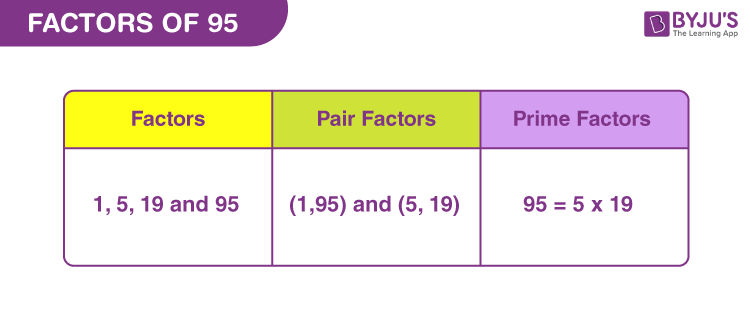# Factors of 95

Factors of 95 divide the original number, completely. These factors are the real numbers. If ‘a’ is the factor of 95, then ‘a’ divides 95 into equal parts. For example, 4 is the factor of 8, because 4 divides 8 into two equal parts (8/4 = 2). Thus, we can see, after division by the factor, we get the whole number as the quotient and the remainder is zero.

Now, let us find what are the numbers that are factors of 95 along with its pair factors and prime factors.## How to Find the Factors of 95?

Factors of 95 are the integers that divide the original number, wholly. Since 95 is a composite number, therefore, it will have more than two factors. Thus, all the factors will divide 95 into equal parts.

To calculate the factors, we need to start dividing 95 by the smallest natural number, i.e., 1.

• 95 ÷ 1 = 95
• 95 ÷ 5 = 19
• 95 ÷ 19 = 5
• 95 ÷ 95 = 1

Thus, the factors of 95 are 1, 5, 19 and 95.

## Pair Factors of 95

The product of those factors that result in the original number is called pair factors. Thus, pair factors of 95 are those two factors, the product of which results in 95.

• 1 × 95 = 95
• 5 × 19 = 95

Therefore, the pair factors are (1, 95) and (5, 19).

Similarly, considering the negative pair factors, we get;

• -1 × -95 = 95
• -5 × -19 = 95

Therefore, the negative pair factors are (-1, -95) and (-5, -19).

## Prime Factorisation of 95

Prime factors are the prime numbers that can divide 95 equal parts. To find these prime factors, we can use the prime factorization method, where each time we need to divide 95 by a prime number unless we get 1.

List of prime numbers:

 2, 3, 5, 7, 11, 13, 17, 19, 23, 29, 31, 37, 41, 43, 47, 53, 59, 61, 67, 71, 73, 79, 83, 89

Step 1: Since 95 is an odd number, we cannot divide it by 2. Also, it is not divisible by 3. At the unit place, we have 5, so it is divisible by 5. 95 divided by 5

95/5 = 19

Step 2: Now 19 is itself a prime number, as we can see from the list. Thus,

19/19 = 1

Therefore,

 Prime factorisation of 95 = 5 x 19

## Solved Examples

Q.1: Rekha has Rs. 95 in her purse. She bought 5 chocolates and is left with zero rupees now. What is the cost of each chocolate then?

Solution: Given,

Total rupees Rekha has in her purse = Rs. 95

Number of chocolates she bought for Rs. 95 = 5

Therefore,

Cost of each chocolate = 95/5 = 19

Thus, each chocolate cost Rs. 19.

Q.2: Find the sum of all the factors of ninety-five.

Solution: The factors of 95 are 1, 5, 19 and 95.

Sum = 1 + 5 + 19 + 95 = 120

Therefore, 120 is the required sum.

Q.3: What is the greatest common factor of 105 and 95?

Answer: Let us write the factors of both the numbers.

105 → 1, 3, 5, 7, 15, 21, 35, 105

95 → 1, 5, 19 and 95

Hence, the greatest common factor is 5.

## Frequently Asked Questions on Factors of 95

### 1. What are the factors of 95?

Answer: There are a total of four factors of 95, they are 1, 5, 19 and 95.

### 2. What are the multiples of 95?

Answer: The first 10 multiples of 95 are 95 + 190 + 285 + 380 + 475 + 570 + 665 + 760 + 855 + 950.

### 3. What are the common factors of 94 and 95?

Answer: The factors of 94 are 1, 2, 47, 94 and factors of 95 are 1, 5, 19, 95. Therefore, only 1 is the common factor.

### 4. 95 is a prime number. True or False?

Answer: False. 95 is a composite number because it has a total of 4 factors.

### 5. What is 95 as a product of primes?

Answer: 95 as a product of primes is represented by:

95 = 5 x 19.

### 6. What is the average of factors of 95?

Answer: Average = Sum of all the factors divided by the total number of factors.

Average = (1 + 5 + 19 + 95)/4

Average = 120/4 = 30

Therefore, 30 is the required average.